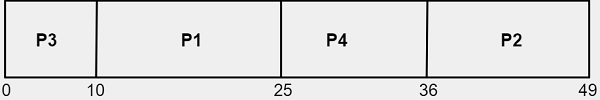# C++ Program for Priority Scheduling

We are given with the n number of processes i.e. P1, P2, P3,.......,Pn with their corresponding burst times and priorities associated with each process . The task is to find the average waiting time ,average turnaround time and the sequence of process execution using priority CPU scheduling algorithm.

What is Waiting Time and Turnaround Time?

Turnaround Time is the time interval between the submission of a process and its completion.

Turnaround Time = completion of a process – submission of a process

Waiting Time is the difference between turnaround time and burst time

Waiting Time = turnaround time – burst time

What is Priority Scheduling?

In priority scheduling, every process is associated with a priority ranging from 0-10 where, integer 0 represents the lowest priority and 10 represents the highest priority. Priorities can be defined in two ways i.e. internally and externally. Also, priority scheduling can be either preemptive or nonpreemptive.

In preemptive priority scheduling, scheduler will preempt the CPU if the priority of newly arrived process is higher than the priority of a process under execution.

In nonpreemptive priority scheduling, scheduler will queue the new process at the head of the ready queue.

Disadvantage of using priority scheduling algorithm is indefinite blocking or starvation. There will be a low priority process who might have to wait indefinite for resources because of high priority process which will lead to starvation problem.

## Example

Let’s say there are 4 processes P1, P2, P3 and P4 with their corresponding burst time and priorities associated with each process where 0 represents lowest priority and 10 represents the highest priority.

ProcessBurst TimePriority
P1152
P2130
P3104
P4111

Sequence of executing multiple processes will be represented using gantt chart given below## Algorithm

Start
Step 1-> Make a structure Process with variables pid, bt, priority
Step 2-> In function bool compare(Process a, Process b)
Return (a.priority > b.priority)
Step 3-> In function waitingtime(Process pro[], int n, int wt[])
Set wt = 0
Loop For i = 1 and i < n and i++
Set wt[i] = pro[i-1].bt + wt[i-1]
End
Step 4-> In function turnarround( Process pro[], int n, int wt[], int tat[])
Loop For i = 0 and i < n and i++
Set tat[i] = pro[i].bt + wt[i]
End Loop
Step 5-> In function avgtime(Process pro[], int n)
Declare and initialize wt[n], tat[n], total_wt = 0, total_tat = 0
Call function waitingtime(pro, n, wt)
Call function turnarround(pro, n, wt, tat)
Print “Processes, Burst time, Waiting time, Turn around time"
Loop For i=0 and i<n and i++
Set total_wt = total_wt + wt[i]
total_tat = total_tat + tat[i]
End Loop
Print values of “Processes, Burst time, Waiting time, Turn around time"
Print Average waiting time, Average turn around time
Step 6-> In function scheduling(Process pro[], int n)
Call function sort(pro, pro + n, compare)
Loop For i = 0 and i < n and i++
Print the order.
End Loop
Call function avgtime(pro, n)
Step 7-> In function int main()
Declare and initialize Process pro[] = {{1, 10, 2}, {2, 5, 0}, {3, 8, 1}}
Declare and initialize n = sizeof pro / sizeof pro
Call function scheduling(pro, n)
Stop

## Example

Live Demo

#include<bits/stdc++.h>
using namespace std;
struct Process {
int pid; // Process ID
int bt; // CPU Burst time required
int priority; // Priority of this process
};
// sorting the Process acc. to the priority
bool compare(Process a, Process b) {
return (a.priority > b.priority);
}
void waitingtime(Process pro[], int n, int wt[]) {
// Initial waiting time for a process is 0
wt = 0;
// calculating waiting time
for (int i = 1; i < n ; i++ )
wt[i] = pro[i-1].bt + wt[i-1] ;
}
// Function to calculate turn around time
void turnarround( Process pro[], int n, int wt[], int tat[]) {
// calculating turnaround time by adding
// bt[i] + wt[i]
for (int i = 0; i < n ; i++)
tat[i] = pro[i].bt + wt[i];
}
//Function to calculate average time
void avgtime(Process pro[], int n) {
int wt[n], tat[n], total_wt = 0, total_tat = 0;
//Function to find waiting time of all processes
waitingtime(pro, n, wt);
//Function to find turn around time for all processes
turnarround(pro, n, wt, tat);
//Display processes along with all details
cout << "\nProcesses "<< " Burst time " << " Waiting time " << " Turn around time\n";
// Calculate total waiting time and total turn
// around time
for (int i=0; i<n; i++) {
total_wt = total_wt + wt[i];
total_tat = total_tat + tat[i];
cout << " " << pro[i].pid << "\t\t" << pro[i].bt << "\t " << wt[i] << "\t\t " << tat[i] <<endl;
}
cout << "\nAverage waiting time = " << (float)total_wt / (float)n;
cout << "\nAverage turn around time = " << (float)total_tat / (float)n;
}
void scheduling(Process pro[], int n) {
// Sort processes by priority
sort(pro, pro + n, compare);
cout<< "Order in which processes gets executed \n";
for (int i = 0 ; i < n; i++)
cout << pro[i].pid <<" " ;
avgtime(pro, n);
}
// main function
int main() {
Process pro[] = {{1, 10, 2}, {2, 5, 0}, {3, 8, 1}};
int n = sizeof pro / sizeof pro;
scheduling(pro, n);
return 0;
}

## Output

Order in which processes gets executed
1 3 2
Processes  Burst time  Waiting time  Turn around time
1              10         0              10
3              8          10             18
2              5          18             23

Average waiting time = 9.33333
Average turn around time = 17